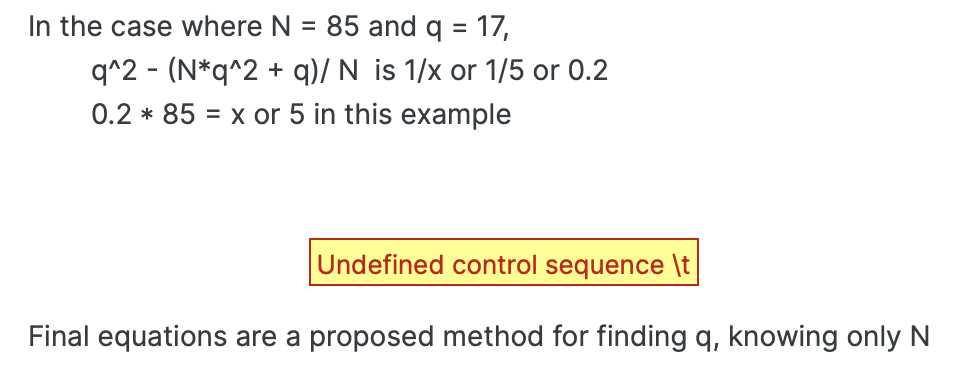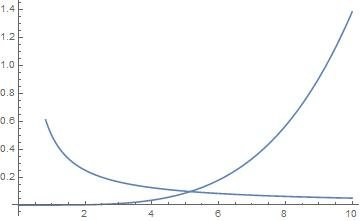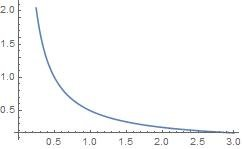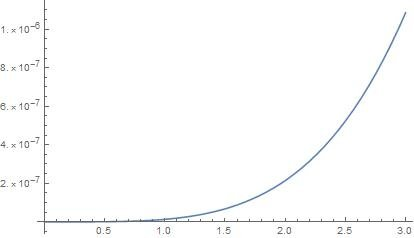# Simple yet interesting.

## Recommended Posts

Posted (edited)
19 hours ago, Trurl said:

I have much more to share.

I am looking forward to a connection to RSA encryption and prime factorisation of large integers.

On 6/20/2021 at 10:50 PM, Trurl said:

Plug and chug

Ok.

I'll try two examples: 8633, 8637. Both are semi primes.

NSolve  [( x^4/((8637)^2 + x)) == 1]
x≈-92.935
x≈92.935

NSolve  [( x^4/((8633)^2 + x)) == 1]
x≈-92.914
x≈92.914

@Trurl Is this helpful for someone looking for the prime factors of the numbers 8633 and 8637? If so, how?

Edited by Ghideon
grammar

• Replies 117
• Created

#### Posted Images

Ok.

I'll try two examples: 8633, 8637. Both are semi primes.

NSolve  [( x^4/((8637)^2 + x)) == 1]

x≈-92.935

x≈92.935

NSolve  [( x^4/((8633)^2 + x)) == 1]

x≈-92.914

x≈92.914

@Trurl Is this helpful for someone looking for the prime factors of the numbers 8633 and 8637? If so, how?

Yes the numbers are too close to estimate. But I still have faith in the Pappy Craylar method. After all 3 < 92 and 3^4/(8637^2+3)=0.000001732

Which Is close to zero where the error is supposed to be near anyway.

Remember when I said we needed to test the logarithmic curve of 3*5; 3*7; 3*11 and so forth to find the error change as 3*Prime number increases?

I know your smart and are looking for holes in the PC method. That is what you are supposed to do. But I know you are smart enough to understand it.

A fix to to great number of possible estimates is to isolate y as x is isolated. Then we will see a better picture of what is happening when SemiPrimes are factored.

##### Share on other sites

20 hours ago, Trurl said:

Yes the numbers are too close to estimate. But I still have faith in the Pappy Craylar method. After all 3 < 92 and 3^4/(8637^2+3)=0.000001732

Which Is close to zero where the error is supposed to be near anyway.

How did you get the number 3? From your formulas or by some other method?

20 hours ago, Trurl said:

I know your smart and are looking for holes in the PC method. That is what you are supposed to do. But I know you are smart enough to understand it.

I do not understand. If that is due to my lack of abilities or lack of valid explanations, I'll let others judge.

And I am still waiting for a connection to RSA encryption and prime factorisation of large integers.

##### Share on other sites

(y^3 * pnp^3 - y^2 * pnp + pnp) / (y^3 * pnp + y^2) = pnp^2

y is now isolated as x was. Ready to simplify.

No, you uncovered a weakness of the PC method. That is that the error varies between 0 and 1. The first SemiPrime was close to 94. I factored the second to be sure. But by working with the equation for years that it could be a smaller Prime factor.

The trouble is without knowing the true error testing numbers less than 94 in the example, narrows the selection but not more useful than division. We need exact results and not an estimate. But does that exist?

3 possible solutions:

• Isolate y and compare to x
• Investigate the curve of the graph of the equation between 0 and 1
• Find a pattern in the error. An error not spanning such a large range.

3^4/(8637^2+3)=0.000001732

3 < 94 but the number of importance is 0.00001732. It causes 3 to be hidden when starting at 1 and not zero.

Questions are good. It is quite possible I am wrong. But I see something or I wouldn’t have put so much effort.

From Wikipedia:

Semiprimes are highly useful in the area of cryptography and number theory, most notably in public key cryptography, where they are used by RSA and pseudorandom number generators such as Blum Blum Shub. These methods rely on the fact that finding two large primes and multiplying them together (resulting in a semiprime) is computationally simple, whereas finding the original factors appears to be difficult. In the RSA Factoring Challenge, RSA Security offered prizes for the factoring of specific large semiprimes and several prizes were awarded. The original RSA Factoring Challenge was issued in 1991, and was replaced in 2001 by the New RSA Factoring Challenge, which was later withdrawn in 2007.

##### Share on other sites

I'm coming to this thread late and trying to make sense of it. @Trurl, your writing style is difficult to parse. You should also learn $\LaTeX$ which will make it easier for others to read your math.

@Trurl correct me if I'm wrong about your work and motivation. This is what I understand you're trying to do. The motivation is to find a method of approximating the smaller prime factor of a semi-prime. So, if $N$ is a semi-prime and $p$ and $q$ are primes such that $q<p$ and $N=pq$, then you want a function $f(x)$ such that there exists a point $(n, f(n))$ where $f(n) \approx q$.

And the reason you want such a function is so if $n$ is known or easy to find, then you limit the search space to find $q$ and you also approximately know the lower bound for $p$ because it must be greater than $q$.

7 hours ago, Ghideon said:

How did you get the number 3? From your formulas or by some other method?

I do not understand. If that is due to my lack of abilities or lack of valid explanations, I'll let others judge.

And I am still waiting for a connection to RSA encryption and prime factorisation of large integers.

I don't think he gets the number 3, it's a counter example.

Edited by FragmentedCurve
##### Share on other sites

\frac {\text {pnp}^3 y^3 - \text {pnp} y^2 + \text {pnp}} {\text {pnp} y^3 + y^2}

Quote

correct me if I'm wrong about your work and motivation. This is what I understand you're trying to do. The motivation is to find a method of approximating the smaller prime factor of a semi-prime. So, if N is a semi-prime and p and q are primes such that q<p and N=pq, then you want a function f(x) such that there exists a point (n,f(n)) where f(n)q.

And the reason you want such a function is so if n is known or easy to find, then you limit the search space to find q and you also approximately know the lower bound for p because it must be greater than q.

Yes that is exactly it. But does it work? I think it works, but does it prove useful?

I call "N": pnp and "p and q": "x and y"

Given N (I call pnp) we estimate x. And in the latest post I am trying to find y, the larger factor.

\frac {\text {pnp}^3 y^3 - \text {pnp} y^2 + \text {pnp}} {\text {pnp} y^3 + y^2}

\frac {\text {pnp}^3 y^3 - \text {pnp} y^2 + \text {pnp}} {\text {pnp} y^3 + y^2}

$\frac {\text {pnp}^3 y^3 - \text {pnp} y^2 + \t ext {pnp}} {\text {pnp} y^3 + y^2}$

##### Share on other sites

The new "y isolated" equation does not work. It was based on the "x isolated" equation, but does not work.

Does anyone see a way to isolate y knowing only pnp?

##### Share on other sites

I don't really know how to answer your questions because they don't really make sense. From what I can gather, the important thing is you want $x>q$. Below I give your conjecture with a simpler function that has the same property along with a proof.

Conjecture

Given the function $f(x)=\frac{x^2}{N}$, if N=pq  is a semiprime and p>q , then p>x>q when f(x)=1 .

Proof

$1=\frac{x^2}{N} \implies \sqrt{N}=x$

Given that p>q , then $p^2>N>q^2 \implies p>\sqrt{N}>q \therefore p>x>q$.

Edited by FragmentedCurve
LaTeX issues
##### Share on other sites

Here are my equations to review. There are quite possibly hundreds of variations. This is what I meant by isolating x and y in the equations. I mean isolated p and q separately, knowing only N. There are 3 equations. I am just getting used to Latex so I hope this is readable. If necessary I will post corrections. But it is these 3 separate equations I want you to test.

p^3 - (p^3*N^2) / (N^2 + p)

$p^3 - \frac {N^2 p^3} {N^2 + p}$

___ ___ ___ ___ ___ ___ ___ ___ ___ ___ ___ ___ ___ ___ ___ ___ ___ _

The above equation should be close to zero, but sometimes the error is closer to 1

(p^3 *N) / (N^2 + p) = fraction
fraction/ q = fraction / (N /p)
Sqrt[fraction / (N /p)] * N = p^2

$\frac{N p^3}{N^2+p}=\text{fraction}$
___________________________________________________________________

The above equations are the second example.

q = Sqrt[(N*q^2 + q)/ N]

q^2 - (N*q^2 + q)/ N  is approximately 0

In the case where N = 85 and q = 17,
q^2 - (N*q^2 + q)/ N  is 1/x or 1/5 or 0.2
0.2 * 85 = x or 5 in this example

$q^2 - \frac {0\text {approximately}\t ext {is}\left (N q^2 + q \right)} {N}$

Final equations are a proposed method for finding q, knowing only N

I have been working with the first equation for awhile. The other 2 are based on the first, but still need tried.

There is nothing fancy here. I am just comparing how numbers divide.

##### Share on other sites

@Trurl there are some Latex errors in your message,  it is not readable. Here is a screenshot of how it looks like:##### Share on other sites

Note: These are 3 separate groups of equations. They are separated by lines.

$p^3 - \frac {N^2 p^3} {N^2 + p}$

___ ___ ___ ___ ___ ___ ___ ___ ___ ___ ___ ___ ___ ___ ___ ___ ___ _

The above equation should be close to zero, but sometimes the error is closer to 1

$\frac{N p^3}{N^2+p}=\text{fraction}$

because q = N/p

$\frac{\text{fraction}}{q}=\frac{\text{fraction}}{\frac{N}{p}}$

$N \sqrt{\frac{\text{fraction}}{\frac{N}{p}}}=p^2$
___________________________________________________________________

The above equations are the second example.

$q = \sqrt {\frac {N q^2 + q} {N}}$

square q

$q^2-\frac{ \left(N q^2+q\right)}{N}$

In the case where N = 85 and q = 17,

$q^2 - (N*q^2 + q)/ N$     is 1/x or 1/5 or 0.2

$0.2 * 85 = x$ or               5 in this example

These are 3 separate groups of equations. They are separated by lines.

Edited by Trurl
##### Share on other sites

On 10/17/2021 at 9:43 PM, Trurl said:

The above equation should be close to zero

Why?

On 10/17/2021 at 9:43 PM, Trurl said:

In the case where N = 85 and q = 17,

q2(Nq2+q)/N

is 1/x or 1/5 or 0.2

What is the purpose? Note that $q^2-\frac{N q^2+q}{N}=-q/N$

##### Share on other sites

Quote

On 10/17/2021 at 3:43 PM,  Trurl said:

The above equation should be close to zero

Why?

Because it is p^2 minus p^2 derived.

Same with second equation. It is just how I derived the equations. Does it work? I don’t know. But I am simply comparing patterns in division.

Obviously you tried test values. Did it work with your values?

i will run more test values. Let me know if you have any more questions or your test values don’t work.

##### Share on other sites

@Ghideon

I know you are not going to like my explanation, but q/N is enough to get an estimate of N. Yes I know it is true for all numbers, but using the reciprocal of p and q helps determine where they are positioned on the number line.

For semi-Primes my hypothesis is that q/N will reduce to 1/p.

Then take 1/p and multiply it by N.

You could argue that it is no more useful than recursive division. However, it does give an estimate of where y lies on the number line. And it could further improve estimates of x when determining how far (N minus p) is from (N minus p calculation).

I know it seems dumb. Why did we divide in the first place if a simple q/N could solve the solution? And it is a high possibility it doesn’t work. But I am saying the simple solution to the semi-Prime factors problem is helpful. That is: not helpful for a beautiful math equation, but helpful when trying to find a hack to defeat a problem that was never a one-way-function from the start.

Clear[pnp, q]

pnp = 85
q = 17

Simplify[(q^2 - (pnp*q^2 + q)/ pnp)]

Out= 85

Out= 17

-(1/5)

___ ___ ___ ___ ___ ___ ___ ___ ___ ___ ___ ___ ___ ___ ___ ___ ___ \
___ ___ ___ ___ ___ ___ __

In:=

Clear[pnp, q]

pnp = 85
q = 19

Simplify[(q^2 - (pnp*q^2 + q)/ pnp)]

Out= 85

Out= 19

-(19/85)

___ ___ ___ ___ ___ ___ ___ ___ ___ ___ ___ ___ ___ ___ ___ ___ ___ \
___ ___ ___ ___ ___ ___ ___

In:=

Clear[pnp, q]

pnp = 293*3
q = 293

Simplify[(q^2 - (pnp*q^2 + q)/ pnp)]

Out= 879

Out= 293

Out= -(1/3)

___ ___ ___ ___ ___ ___ ___ ___ ___ ___ ___ ___ ___ ___ ___ ___ ___ \
___ ___ ___ ___ ___ ___ ___ _

In:=

Clear[pnp, q]

pnp = 293*3
q = 291

Simplify[(q^2 - (pnp*q^2 + q)/ pnp)]

Out= 879

Out= 291

Out= -(97/293)

In:= N[-(97/293)]

In:= -0.3310580204778157* 293*3

Out= -291.

##### Share on other sites

• 2 weeks later...
On 3/3/2021 at 7:15 PM, Trurl said:

Remember I am claiming I can factor semi-Primes and thus RSA cryptography would be no more.

I think that current RSA encryption is quite unaffected by the things presented in this tread.

##### Share on other sites

• 2 weeks later...

You must tell me why RSA is not affected by this thread.

We can agree that RSA was based on the Prime factorization problem. That is factoring Semi-Primes into the Prime factors.

So you must not trust my algebraic equations that estimate where the factors of semi-Primes occur. That is the basis of my work. I think I have stated what they do. But in your test where N=p*q where do you not believe p cannot be found knowing only N?

$$q\sqrt {\frac {\text {pnp}^3} {\text {pnp} q^2 + q}}$$

Edited by Trurl
Latex
##### Share on other sites

On 11/16/2021 at 1:13 AM, Trurl said:

You must tell me why RSA is not affected by this thread.

The security of RSA relies on the practical difficulty of factoring the product of two large prime numbers, the "factoring problem". So far this thread does not contain any intelligible description of a new method for factorisation and certainly no improvements over the current state of the art methods.

No-one seems to understand your approach towards factoring but still there are several members that have explained why it can not work. Five pages into the discussion I’m pretty confident that there will never be any useful description provided.

Equations and associated claims have been dismissed by simple counter examples.

The result is that my current level of confidence in RSA is not changed by anything provided in this thread and my best guess is that my opinion is shared by a. wast majority of users of RSA encryption.

Edited by Ghideon
##### Share on other sites

• 2 weeks later...

Klapaucius, uh um, I mean Ghideon,

You act as there is noting of value in this tread. Sure, breaking RSA would be quite a feat. But you must admit there is no other method that does it. There is no guarantee the Pappy Craylar Method works. And I have moved on to other projects. However, we had an active discussion that may lead to something that might find a different approach.

You see, The Pappy Craylar Method is based on the fact that if you increase the size of one factor you must decrease the other. And since there is only one way the Semi-Prime factors go together, the equation to find the factors eliminates possibilities. Sure, the number of possibilities isn’t only one and sometimes the possibilities are further or closer to zero. But until a solution exists, the method leads to the ability to guess the factors by trial and error.

See my construction of the 2 graphs that intersect at 5. Of course, I already new the answer, but don’t you find the PC method simple yet interesting?

In:= pnp = 85
x = 5

Show[{Plot[(x^4/(pnp^2 + x)), {x, 0, 10}],
Plot[(pnp - (x*Sqrt[(pnp^3/(pnp* x^2 + x))])), {x, 0, 10}]}]

Out= 85

Out= 5In:=

Clear[pnp, x]
pnp = 8637

{Plot[(x^4/(pnp^2 + x)), {x, 0, 3}]
Plot[(pnp - (x*Sqrt[(pnp^3/(pnp* x^2 + x))])), {x, 0, 3}]}

Out= 8637`Does this work for every Semi-Prime? Maybe, maybe not. This is all I have to share. I am moving on to other projects. So, unless some idea comes along, I will not post. Is my last message Simple, yet interesting?

## Create an account

Register a new account